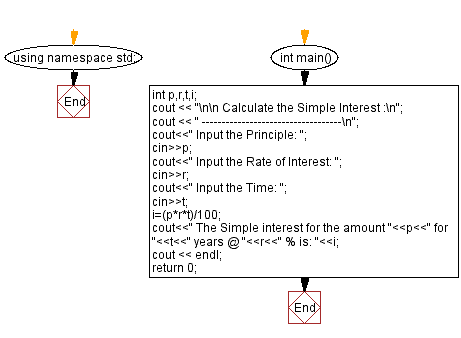﻿ C++ Exercises: Calculate Simple Interest - w3resource

# C++ Exercises: Calculate Simple Interest

## C++ Basic: Exercise-54 with Solution

Write a C++ program to enter P, T, R and calculate Simple Interest.

Sample Solution:

C++ Code :

``````#include<iostream> // Including input-output stream header file
using namespace std; // Using the standard namespace

int main() { // Start of the main function

int p, r, t, i; // Declaring variables to store principle, rate, time, and interest

cout << "\n\n Calculate the Simple Interest :\n"; // Displaying the purpose of the program
cout << " -----------------------------------\n";

cout << " Input the Principle: "; // Prompting the user to input the principle amount
cin >> p; // Taking input of the principle amount from the user

cout << " Input the Rate of Interest: "; // Prompting the user to input the rate of interest
cin >> r; // Taking input of the rate of interest from the user

cout << " Input the Time: "; // Prompting the user to input the time period
cin >> t; // Taking input of the time period from the user

i = (p * r * t) / 100; // Calculating the simple interest using the formula: PRT / 100

cout << " The Simple interest for the amount " << p << " for " << t << " years @ " << r << " % is: " << i; // Displaying the calculated simple interest

cout << endl; // Displaying an empty line for better readability
return 0; // Returning 0 to indicate successful program execution
}
``````

Sample Output:

``` Calculate the Simple Interest :
-----------------------------------
Input the Principle: 20000
Input the Rate of Interest: 10
Input the Time: 1.5
The Simple interest for the amount 20000 for 1 years @ 10 % is: 2000
```

Flowchart:C++ Code Editor:

What is the difficulty level of this exercise?

﻿